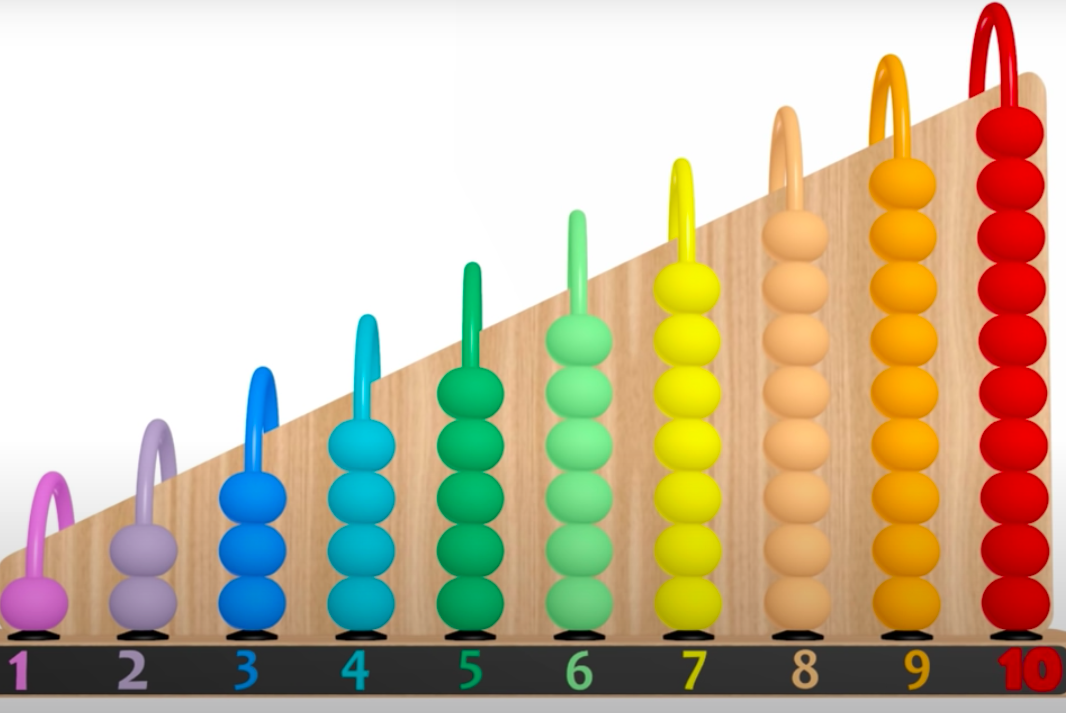# A step by step guide to the Counting Sort algorithmA detailed guide to the counting sort algorithm with implementations in C++, C# and Python.

While all comparison-based algorithms have a time complexity of O(nlogn) to sort an array of n elements, there are sorting algorithms running in linear time provided that some assumptions are verified.

# A quick introduction to the ring buffer data structureThis post presents the Ring Buffer data structure, showing a possible implementation in C++ using templates.

Ring buffers (also known as circular buffers) are fixed-size buffers that works as if the memory were contiguous and circular.

# Recursion vs iterationThis post is about using recursion vs using iteration.

The most straightforward application of recursion is to replace iteration using a recursive function instead of for/while loops. Such recursive functions allow to iterate over data structures, taking as parameter the index that would be used in a for loop. As an example, let's consider the following code printing a c++ vector: